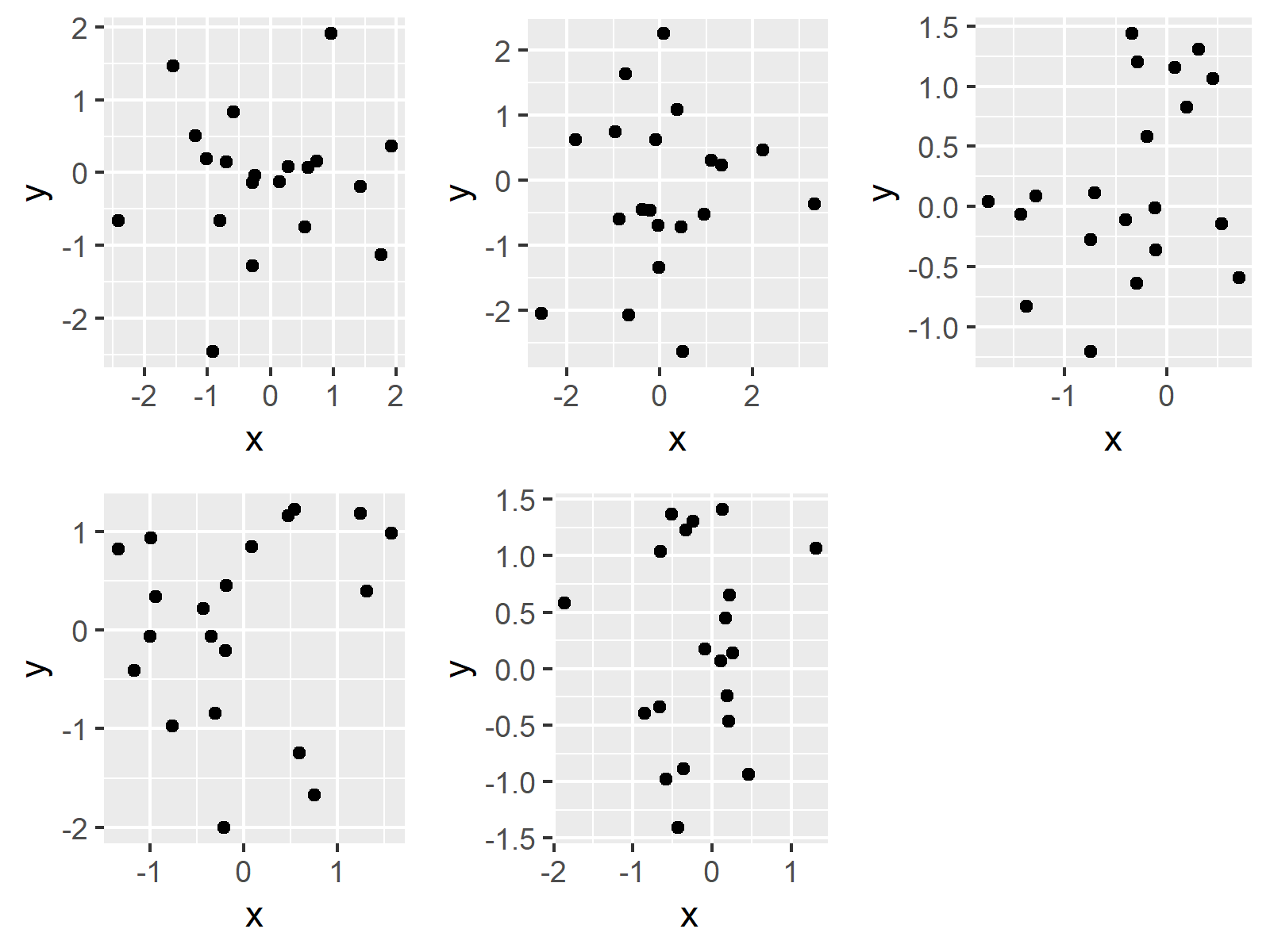# Arrange List of ggplot2 Plots in R (Example)

On this page you’ll learn how to draw a list of ggplot2 plots side-by-side in a plot layout using the R programming language.

Let’s take a look at some R codes in action.

## Example Data, Packages & Basic Graph

The data below is used as basement for this R programming tutorial:

```set.seed(47499)                                                   # Create example data
data <- data.frame(x = rnorm(100),
y = rnorm(100),
group = rep(LETTERS[1:5],
times = 20))
#             x          y group
# 1 -0.91956298 -2.4521731     A
# 2 -0.03343599 -0.6978976     B
# 3 -0.74917821 -0.2750820     C
# 4 -0.30489141 -0.8415968     D
# 5 -0.65035229  1.0372558     E
# 6 -0.28318237 -1.2768368     A```

Have a look at the previous output of the RStudio console. It shows that our example data contains three columns – Two numeric variables that will be drawn in the plots later on and a grouping variable defining which value will be drawn in which plot.

For the following tutorial, I also have to install and load the ggplot2 package to R:

```install.packages("ggplot2")                                       # Install ggplot2 package

Now, we can create several ggplot2 plots of the data…

```ggp1 <- ggplot(data[data\$group == "A", ], aes(x, y), col = 1) + geom_point()  # Create plots
ggp2 <- ggplot(data[data\$group == "B", ], aes(x, y), col = 2) + geom_point()
ggp3 <- ggplot(data[data\$group == "C", ], aes(x, y), col = 3) + geom_point()
ggp4 <- ggplot(data[data\$group == "D", ], aes(x, y), col = 4) + geom_point()
ggp5 <- ggplot(data[data\$group == "E", ], aes(x, y), col = 5) + geom_point()```

…and store them in a list of plots:

`plot_list <- list(ggp1, ggp2, ggp3, ggp4, ggp5)                   # Store plots in list`

## Example: Draw List of Plots Using do.call & grid.arrange Functions

In this Example, I’ll explain how to use the do.call and grid.arrange functions to draw all of our plots side-by-side on the same page. First, we need to install and load the gridExtra package, which is containing the grid.arrange function:

```install.packages("gridExtra")                                     # Install gridExtra package

Now, we can draw our list of graphics in a grid as shown below:

`do.call("grid.arrange", c(plot_list, ncol = 3))                   # Apply do.call & grid.arrange`The output of the previously shown R syntax is shown in Figure 1: A grid of ggplot2 scatterplots.

## Video, Further Resources & Summary

The ggplot2 package has of course much more of useful and awesome functions and opportunities when it comes to data visualization in R. May you have a look at the following introduction video on my my YouTube channel.

Please accept YouTube cookies to play this video. By accepting you will be accessing content from YouTube, a service provided by an external third party.If you accept this notice, your choice will be saved and the page will refresh.

In addition, you may want to have a look at the related tutorials of this homepage. Some tutorials are shown here.

To summarize: In this R tutorial you learned how to position multiple ggplot2 graphs using grid.arrange in a composition of graphics. You may use this code to basically arrange and arbitrary number of ggplot2 plots. Please let me know in the comments section, in case you have additional questions.

Subscribe to the Statistics Globe Newsletter